### Skeleton-Intrinsic Symmetrization of Shapes

Computer Graphics Forum (Proceedings of Eurographics 2015)

Qian Zheng1          Zhuming Hao1          Hui Huang1*          Kai Xu1          Hao Zhang2          Daniel Cohen-Or3          Baoquan Chen4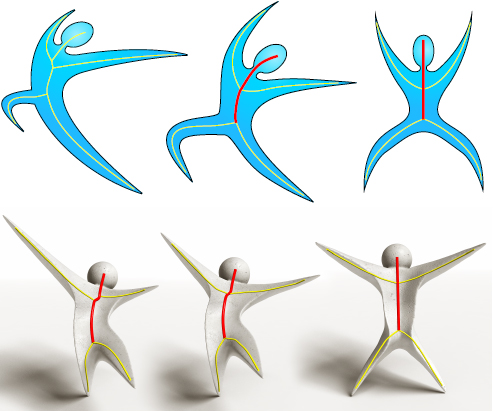Figure 1: Asymmetric shapes (left) are intrinsically symmetrized (middle) about their backbones (red), which facilitates further extrinsic symmetrization (right).

Abstract

Enhancing the self-symmetry of a shape is of fundamental aesthetic virtue. In this paper, we are interested in recovering the aesthetics of intrinsic reflection symmetries, where an asymmetric shape is symmetrized while keeping its general pose and perceived dynamics. The key challenge to intrinsic symmetrization is that the input shape has only approximate reflection symmetries, possibly far from perfect. The main premise of our work is that curve skeletons provide a concise and effective shape abstraction for analyzing approximate intrinsic symmetries as well as symmetrization. By measuring intrinsic distances over a curve skeleton for symmetry analysis, symmetrizing the skeleton, and then propagating the symmetrization from skeleton to shape, our approach to shape symmetrization is skeleton-intrinsic. Specifically, given an input shape and an extracted curve skeleton, we introduce the notion of a backbone as the path in the skeleton graph about which a self-matching of the input shape is optimal. We define an objective function for the reflective self-matching and develop an algorithm based on genetic programming to solve the global search problem for the backbone. The extracted backbone then guides the symmetrization of the skeleton, which in turn, guides the symmetrization of the whole shape. We show numerous intrinsic symmetrization results of hand drawn sketches and artist-modeled or reconstructed 3D shapes, as well as several applications of skeleton-intrinsic symmetrization of shapes.

Overview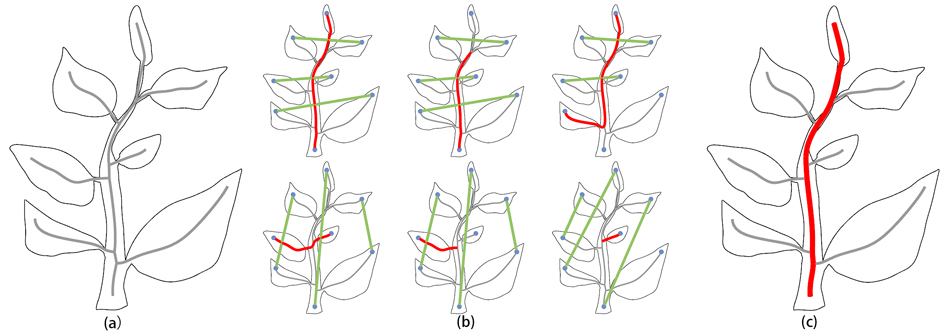Figure 2: Backbone detection: given a shape with an automatically extracted skeleton (a), red curves in (b) represent some potential backbone candidates, where green lines denote the established self-matching between end nodes (blue dots) about the candidate; the red path in (c) is our detected backbone with the optimal self-matching.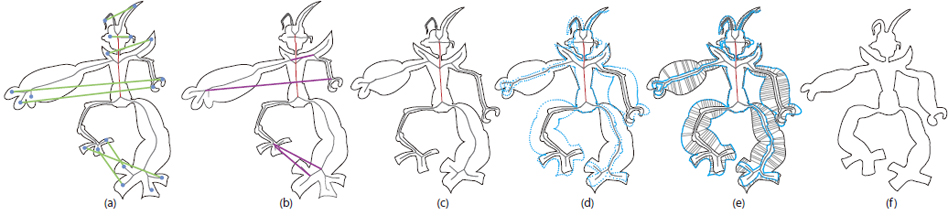Figure 3: Backbone-guided symmetrization: having obtained the backbone and the correspondence between end nodes (a), we first estimate the correspondences between junction nodes (the purple lines in b), and then symmetrize the skeleton about the backbone while deforming its skin accordingly (c). Note that unmatched skeleton segments and their associated surface (the dot lines in b) are simply discarded. The skin is then duplicated and reflected (blue dots in d). The two skins are correlated by matching each point on one skin to the nearest point on the other (e). The final intrinsically symmetric shape is simply the average between these two skins (f).

Results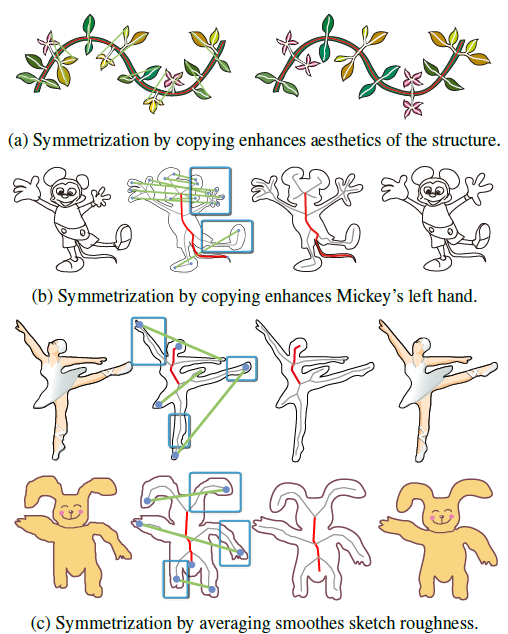Figure 4: Skeleton-intrinsic symmetrization of 2D figures and sketches for beautification and enhancement. In each row, input and output are at the two ends. Middle two figures show skeletons and symmetrized skeletons. Extracted symmetric endpoint pairs are linked by green lines.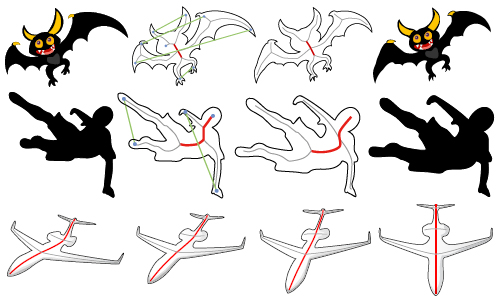Figure 5: Symmetrization of 2D shapes under strong perspective. In the first two rows, the left two images show input, one with skeleton and detected backbone. The right two images show the symmetrized results. Last row shows a series of gradually symmetrized (both intrinsically and then extrinsically) airplanes, providing the illusion of a“rotation”.

Acknowledgments

The authors would like to thank all the reviewers for their valuable comments and feedback. This work is supported in part by grants from NSFC (61202224, 61331018, 61272327, 61202333), National 973 Program (2014CB360503), National 863 Program (2013AA01A604), Shenzhen Innovation Program (CXB201104220029A, KQCX20120807104901791, ZD201111080115A, JSGG20130624154940238), CPSF China (2012M520392), NSERC (611370 and 293127) and the Israel Science Foundation.

BibTex

@ARTICLE{CGF:CGF12559,
author = {Zheng, Qian and Hao, Zhuming and Huang, Hui and Xu, Kai and Zhang, Hao and Cohen-Or, Daniel and Chen, Baoquan},
title = {Skeleton-Intrinsic Symmetrization of Shapes},
journal = {Computer Graphics Forum},
volume = {34},
number = {2},
pages = {275--286},
year = {2015},
}